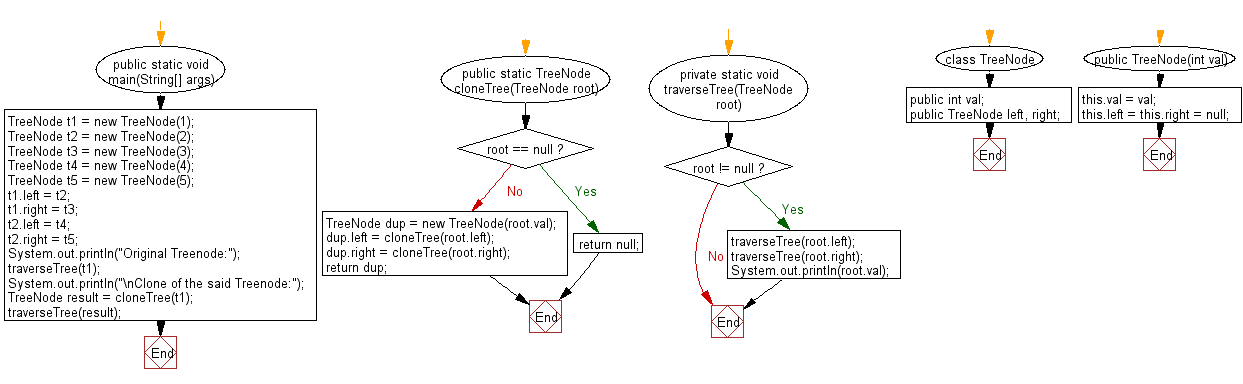﻿ Java programming exercises: Get a new binary tree with same structure and same value of a given binary tree - w3resource# Java Exercises: Get a new binary tree with same structure and same value of a given binary tree

## Java Basic: Exercise-177 with Solution

Write a Java program to get a new binary tree with same structure and same value of a given binary tree.

Sample Solution:

Java Code:

``````import java.util.*;

public class Solution {
public static void main(String[] args) {
TreeNode t1 = new TreeNode(1);
TreeNode t2 = new TreeNode(2);
TreeNode t3 = new TreeNode(3);
TreeNode t4 = new TreeNode(4);
TreeNode t5 = new TreeNode(5);
t1.left = t2;
t1.right = t3;
t2.left = t4;
t2.right = t5;
System.out.println("Original Treenode:");
traverseTree(t1);
System.out.println("\nClone of the said Treenode:");
TreeNode result = cloneTree(t1);
traverseTree(result);
}
public static TreeNode cloneTree(TreeNode root) {
if (root == null) {
return null;
}
TreeNode dup = new TreeNode(root.val);
dup.left = cloneTree(root.left);
dup.right = cloneTree(root.right);
return dup;
}

private static void traverseTree(TreeNode root) {
if (root != null) {
traverseTree(root.left);
traverseTree(root.right);
System.out.println(root.val);
}

}
}
class TreeNode {
public int val;
public TreeNode left, right;

public TreeNode(int val) {
this.val = val;
this.left = this.right = null;
}
}
```
```

Sample Output:

```Original Treenode:
4
5
2
3
1

Clone of the said Treenode:
4
5
2
3
1
```

Flowchart:Java Code Editor:

What is the difficulty level of this exercise?

﻿

## Java: Tips of the Day

Parsing dates:

```import java.io.*;
import java.util.*;
import java.text.*;

String s = "2001/09/23 14:39";

SimpleDateFormat formatter = new SimpleDateFormat ("yyyy/MM/dd H:mm");
Date d = formatter.parse(s, new ParsePosition(0));
```